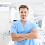ONLY FOR GENIUS

From the above two pictures, can you find out the height of the table?

In the first image, when the cat is on top of the table, the height from top of tortoise to top of cat is 170 cm

In the second image, when the tortoise is on the table, the height from top of tortoise to top of cat is 130 cm.

From the above two equations, can you calculate the height of table?

150 cm is the height of the table.

Explanation
There are two methods through which you can find the height of the table.

METHOD 1:
Equation 1: Cat + Table - Tortoise = 170
Equation 2: Tortoise + Table - Cat = 130

Cat + Table - Tortoise + Tortoise + Table - Cat = 300
=> 2 Table = 300
=> Table = 150

METHOD 2
If you place the right side table on top of the left side table, then the heights of cats will coincide. So the total height of 2 tables will be 300 and so the height of one table is 150 cms.

#### 1 comment:

1.Nicely explained.

George Egbuonu